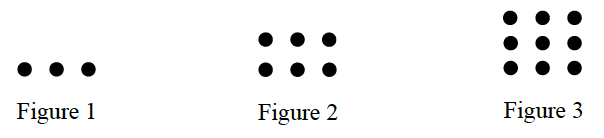### Home > ACC6 > Chapter 4 Unit 4 > Lesson CC1: 4.2.2 > Problem4-59

4-59.

Study the pattern below. Sketch and label the fourth and fifth figures. Then predict how many dots will be in the $100^{th}$ figure. Write an expression you can use to determine the number of dots in any figure. Homework Help ✎In this pattern, you begin with $3$ dots and add $3$ more with each figure.